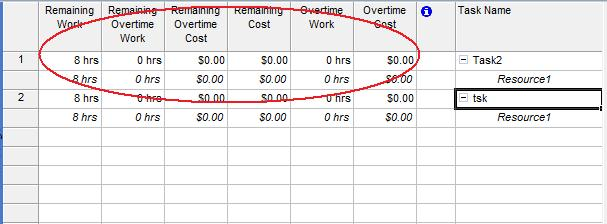# Handling Overtime, Remaining Costs and Work Values

Contents
[ ]

## Handling Overtime, Remaining Costs and Work

The ResourceAssignment class exposes a number of properties for handling an assignment’s overtime, remaining costs and work:

• OvertimeCost represents the sum of the actual and remaining overtime costs of an assignment (decimal).
• OvertimeWork represents the scheduled overtime work for an assignment (TimeSpan).
• RemainingCost represents the remaining projected cost for completing an assignment (decimal).
• RemainingOvertimeCost represents the remaining projected overtime cost for completing an assignment (decimal).
• RemainingWork represents the scheduled remaining work for an assignment (TimeSpan).
• RemainingOvertimeWork represents the scheduled remaining overtime work for an assignment (TimeSpan).

To see assignment overtime, remaining cost and work in Microsoft Project:

1. On the Task Usage screen, select the Insert menu, then Column.